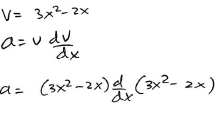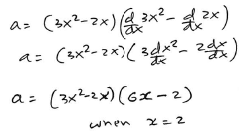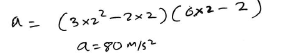Courses

# Integration, Differentiation, Errors & Significant Figures MCQ Level - 1 (Test - 2)

## 25 Questions MCQ Test | Integration, Differentiation, Errors & Significant Figures MCQ Level - 1 (Test - 2)

Description
This mock test of Integration, Differentiation, Errors & Significant Figures MCQ Level - 1 (Test - 2) for Class 11 helps you for every Class 11 entrance exam. This contains 25 Multiple Choice Questions for Class 11 Integration, Differentiation, Errors & Significant Figures MCQ Level - 1 (Test - 2) (mcq) to study with solutions a complete question bank. The solved questions answers in this Integration, Differentiation, Errors & Significant Figures MCQ Level - 1 (Test - 2) quiz give you a good mix of easy questions and tough questions. Class 11 students definitely take this Integration, Differentiation, Errors & Significant Figures MCQ Level - 1 (Test - 2) exercise for a better result in the exam. You can find other Integration, Differentiation, Errors & Significant Figures MCQ Level - 1 (Test - 2) extra questions, long questions & short questions for Class 11 on EduRev as well by searching above.
QUESTION: 1

### If the length of rod A is (3.25 ± 0.01) cm and that of B is (4.19 ± 0.01) cm, then the rod B is longer than rod A by

Solution: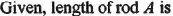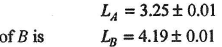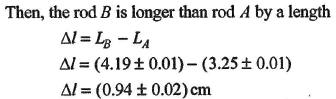QUESTION: 2

### A wire has a mass 0.3 ± 0.003g, radius 0.5 ± 0.005 mm and length 6 ± 0.06 cm. The maximum percentage error in the measurement of its density is 1

Solution: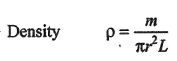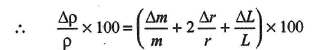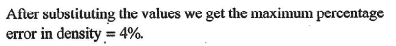QUESTION: 3

### A cube has a side of length 1.2 x 10-2 m. Calculate its volume.

Solution: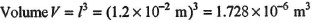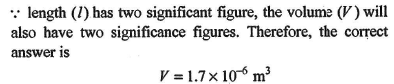QUESTION: 4

If L = 2.331 cm, B - 2.1 cm, then L + B is equal to

Solution: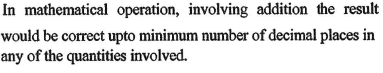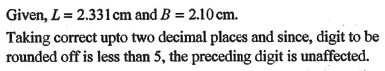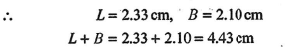QUESTION: 5

A plate has a length (5 ± 0.1) cm and breadth (2 ± 0.01) cm. Then the area of the plate is

Solution:
QUESTION: 6

The number of significant figures in all the given numbers 25.12, 2009, 4.156 and 1.217 x 10-4 is

Solution: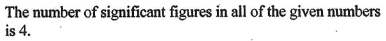QUESTION: 7

An obj ect, m oving with a speed of 6.25 m /s, is decelerated at a rate given by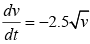where v is the instantaneous speed. The time taken by the object, to come to rest, would be

Solution: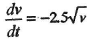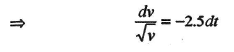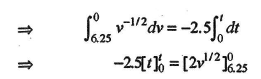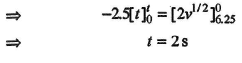QUESTION: 8

The position of a particle x (in metre) at a time t second is given by the relation r  = (3tiˆ - t 2 ˆj + 4kˆ) . Calculate the magnitude of velocity of the particle after 5s. the magnitude of velocity of the particle after 5s.

Solution: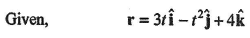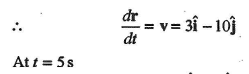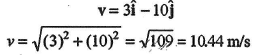QUESTION: 9

A particle moves along with x-axis. The position x of particle with respect to time t from origin given by x = b0 + b1t + b2 t2. The acceleration of particle is

Solution: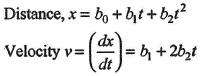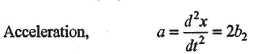QUESTION: 10

The acceleration a of a particle starting from rest varies with time according to relation α = αt + β . The velocity of the particle after a time t will be

Solution: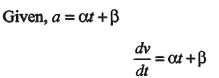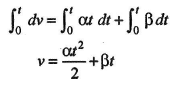QUESTION: 11

A particle moves along a straight line such that its position x at any time t is x = 6t2 -t3. Where x in metre and t is in second, then

Solution: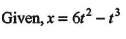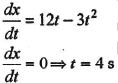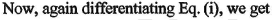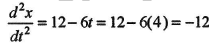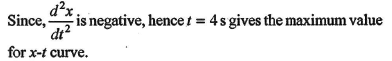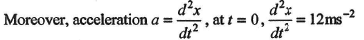QUESTION: 12

Displacement (x) of a particle is related to time (t) as x = at + bt2 - ct3 where a,b and c are constants of the motion. The velocity of the particle when its acceleration is zero is given by

Solution: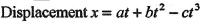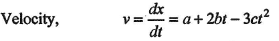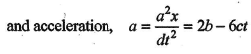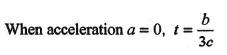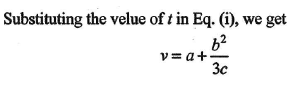QUESTION: 13

Acceleration of a body when displacement equation is 3s = 9t + 5t2 is

Solution: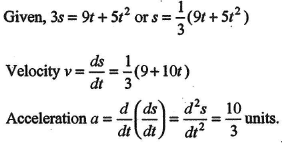QUESTION: 14

The velocity of particle is v = v0 + gt + ft2. If its position is x = 0 at t = 0, then its displacement after unit time (t = 1) is

Solution: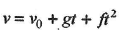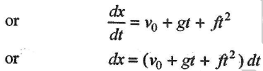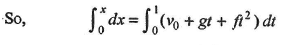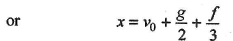QUESTION: 15

The coordinates of a moving particle at any time t are given by x = αt3 and y = βt3. The speed of particle at time t is given by

Solution: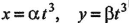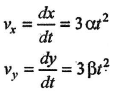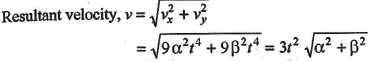QUESTION: 16

A particle located at x = 0 at time t = 0, starts moving along the position x-direction with a velocity v that varies as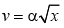.The displacement of the particle varies with time as

Solution: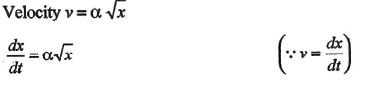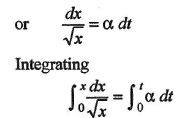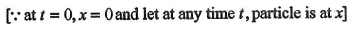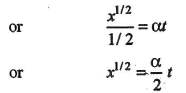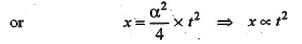QUESTION: 17

The displacement of particle is given by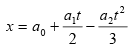What is its acceleration?

Solution: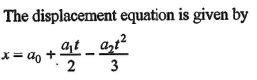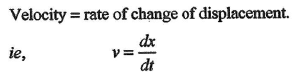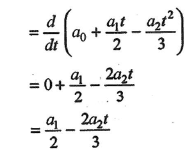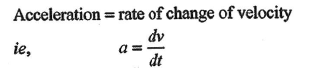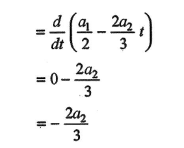QUESTION: 18

The relation between time t and distance x is t = ax2 + bx, where a and b are constants. The acceleration is

Solution: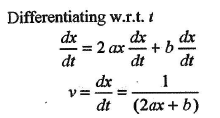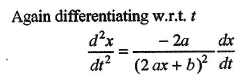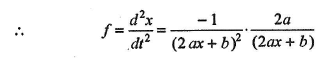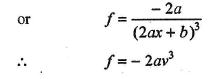QUESTION: 19

A particle moves along x - axis as x = 4 (t - 2) + a (t - 2)2 Which of the following is true?

Solution: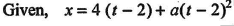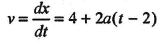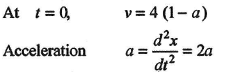QUESTION: 20

If the velocity of a particle is given by v = (180 -16x)1/2 ms-1, then its acceleration will be

Solution: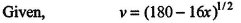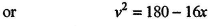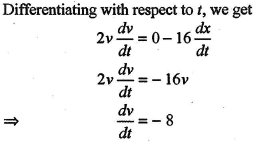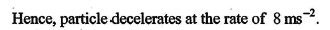QUESTION: 21

A particle moves along x-axis in such a way that its coordinate (x) varies with time t according to the expression x = 2 -5t + 6t2 m, the initial velocity of the particle is

Solution: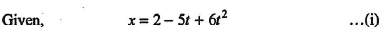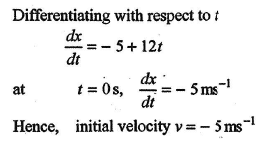QUESTION: 22

A particle moves along a straight line such that its displacement at any time t is given by s = t3 - 6t2 +3t + 4. The velocity when its acceleration is zero is

Solution: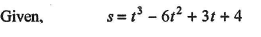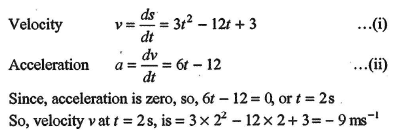QUESTION: 23

The displacement s of a particle is proportional to the first power of time t, i.e.,s α t; then the acceleration of the particle is

Solution: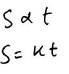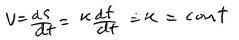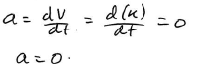QUESTION: 24

The displacement x of particle moving in one dimension under the action of a constant force is related to time t by the equation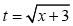, where x is in metres and t is in seconds, Find the displacement of the particle when its velocity is zero

Solution: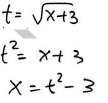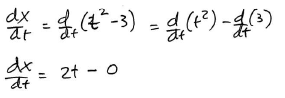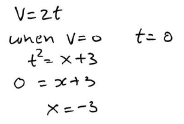QUESTION: 25

For motion of an object along the x-axis, the velocity v depends on the displacement x as v = 3x2 - 2x, then what is the acceleration at x = 2m.

Solution: